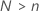# Methods in Analyze Binary Response for Definitive Screening Design

## Factor/covariate pattern

Describes a single set of factor/covariate values in a data set. Minitab calculates event probabilities, residuals, and other diagnostic measures for each factor/covariate pattern.

For example, if a data set includes the factors gender and race and the covariate age, the combination of these predictors may contain as many different covariate patterns as subjects. If a data set only includes the factors race and sex, each coded at two levels, there are only four possible factor/covariate patterns. If you enter your data as frequencies, or as successes, trials, or failures, each row contains one factor/covariate pattern.

## Design matrix

First, Minitab creates a design matrix from the factors and the model that you specify. The columns of this matrix represent the terms in the model. Then, Minitab adds additional columns for the constant term, blocks, and higher-order terms to complete the design matrix for the model in the analysis.

### Designs with all continuous factors

The form of the design matrix depends on whether a conference matrix exists with the same number of rows as factors. When this criterion is true, the columns of the design matrix that represent the factors have this form:
Where C is an n× n conference matrix with elements {-1, 0, 1} that satisfies this property:
When a conference matrix of the correct size does not exist, the columns that represent the factors are a subset of a larger conference matrix:
Where A is an N× n matrix with elements {-1, 0, 1} that satisfies this property:

The full design matrix contains columns besides the columns that represent factors. The design matrix contains a column of 1s for the constant term. The full design matrix also includes columns that represent any square or interaction terms in the model.

### Designs with categorical factors

For a design that includes categorical factors, Minitab replaces the single center point row in the design matrix with 2 pseudo center points. If the design has only 1 categorical factor, only two possible pseudo center points exist, so both points are in the design.

In the case where the design has more than 2 categorical factors, Minitab uses an iterative algorithm to select 2 pseudo center points to include. The algorithm seeks to minimize the variance of the regression coefficients for the linear effects in the model.

### Notation

TermDescription
CA conference matrix
0'A row of zeroes in a matrix that represent a center point run
Inthe n × n identity matrix
AA matrix that is a subset of a conference matrix with N rows and n columns whereNThe number of rows in the subset of the columns from the conference matrix
nThe number of factors in a design
By using this site you agree to the use of cookies for analytics and personalized content.  Read our policy